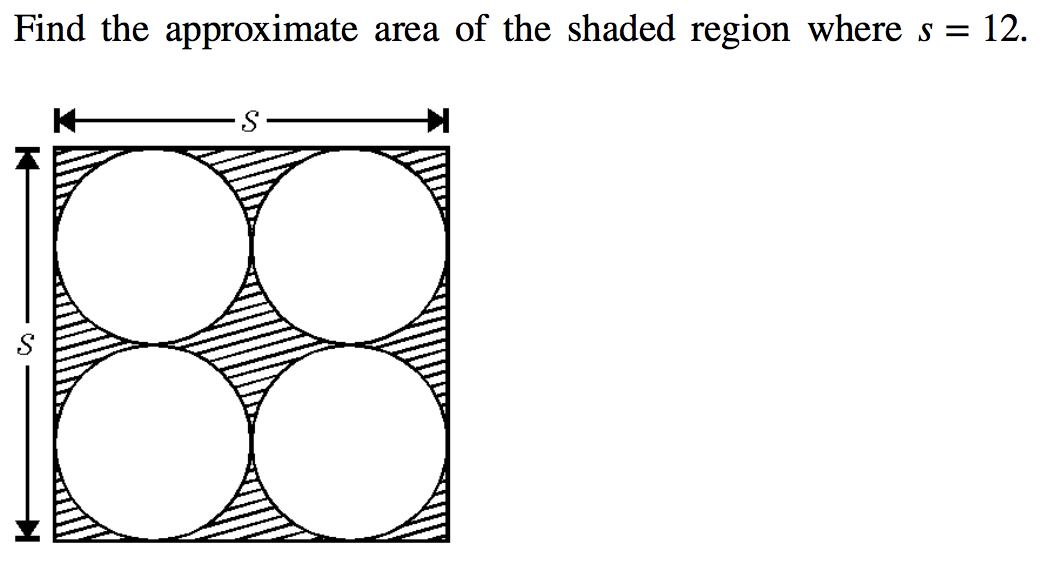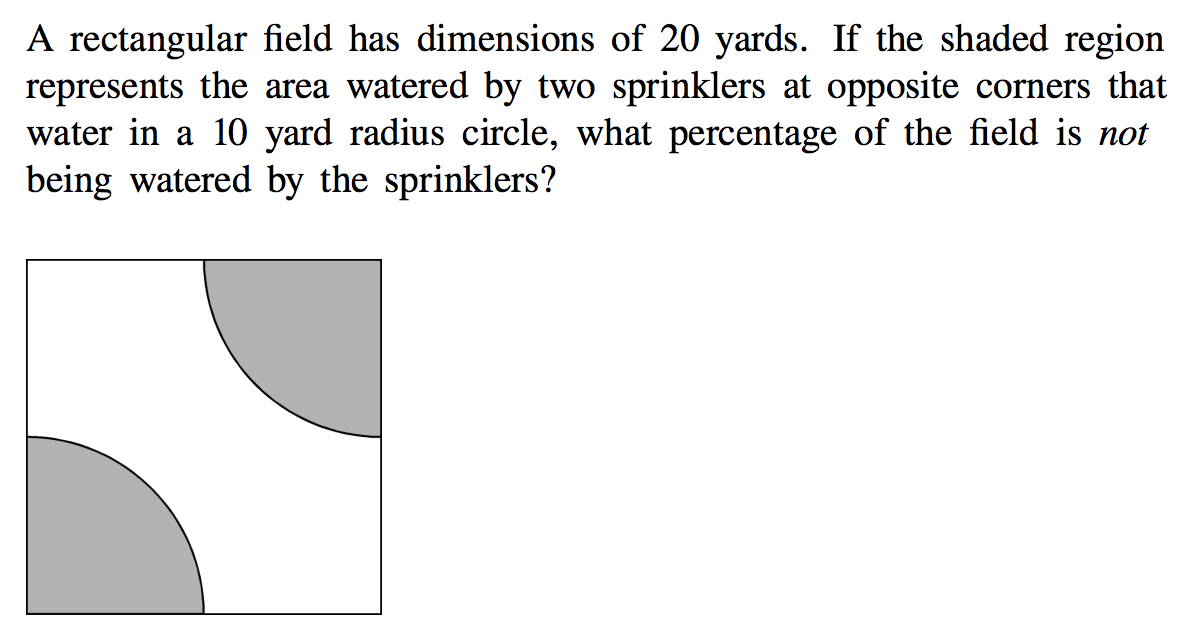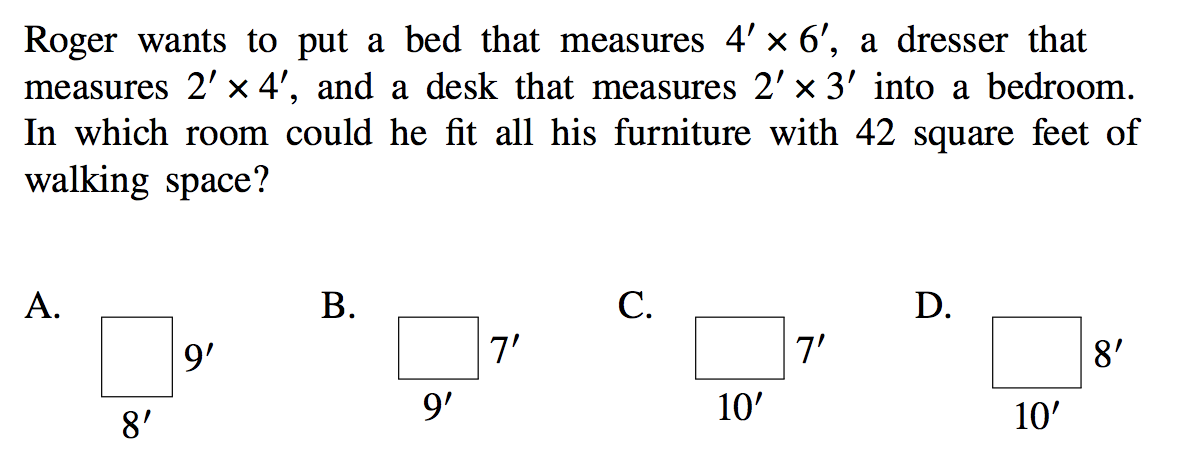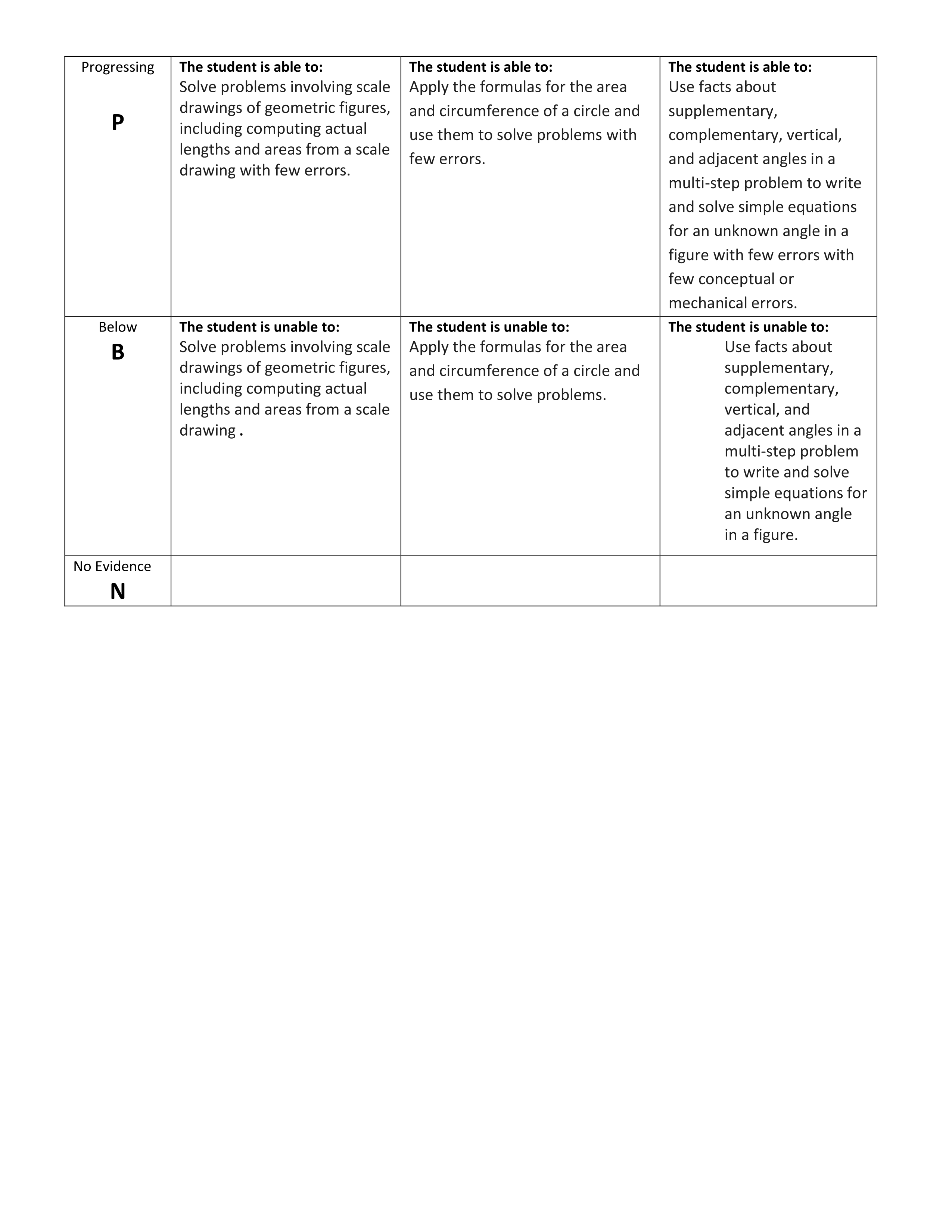Geometry - Summative Assessment - 7.3 - 7.4
starstarstarstarstarstarstarstarstarstar
by Felix Gabathuler
| 13 Questions1
2
1
1
A
B
C
D
2
1
What is the measure of angle m?3
4
5
3
1
Answer yes or no. If you answer yes, tell how many servings and what angle each would be.
4
1
What is the measure of the other acute angle?
5
1
A
B
C
D6
7
8
6
1
Determine the actual area of her room in feet squared. (Do not include units please; round to the nearest tenth if needed)
7
1
What will be the length of the new computer lab?
8
1
Round to the nearest tenth.9
1
Find the approximate area of shaded region above where s = 12. Do not include units in your answer and round to the nearest hundredth if needed.10
1
What percentage of the field is NOT being watered by the sprinklers above? Round your percentage to the nearest hundredth if needed.Try the questions below that will really stretch your skills in Geometry. These are the Exemplary questions that can earn you an E on your summative assessment. Answer 2 of the 3 questions correctly to earn an E! Good luck!11
1
In which room could he fit all his furniture with 42 square feet of walking space?
A
B
C
D12
1
Which expression correctly gives the area of the shaded region above?
A
B
C
D
13
1
A square with an area of 196 inches squared is reduced by a factor of one-half. How long are the new square’s sides?

Below, draw the before and after square with dimensions labeled.